DavidK

•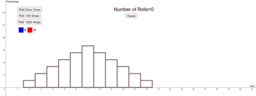Empirical and Theoretical Histograms

Activity

DavidK

•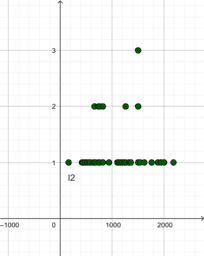Section 2 Descriptive Statistics

Book

DavidK

•Medical Tests: Sensitivity, Specificity, etc

Activity

DavidK

•Probability Collection Book

Book

DavidK

•Introduction to Probability

Book

DavidK

•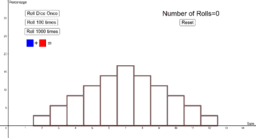The Law of Large Numbers

Activity

DavidK

•Empirical and Theoretical Histograms

Activity

DavidK

•Arctan Range and angle

Activity

DavidK

•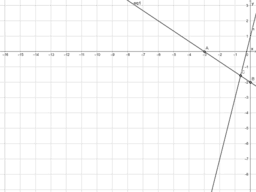Solving System of Equations Substitution

Activity

DavidK

•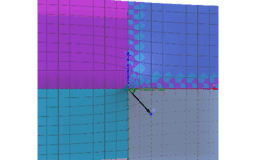arctan and arctan2 functions 3D

Activity

DavidK

•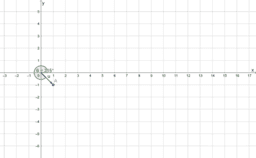arctan and arctan2 functions

Activity

DavidK

•arctan function and range

Activity

DavidK

•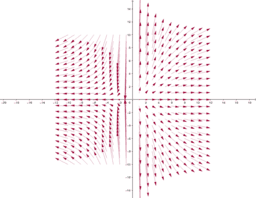Vector Field 2D on Grid

Activity

DavidK

•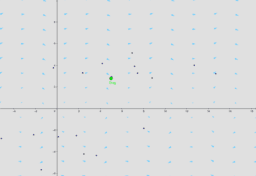Velocity Field and Flow Line Trace

Activity

DavidK

•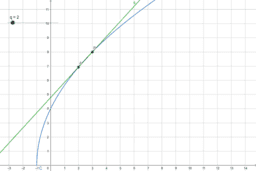Cost Function Non-Linear Tangent Secant

Activity

DavidK

•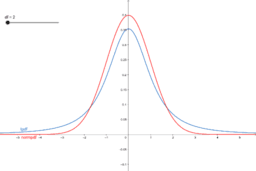Student t-Distribution Compared with Normal Dist

Activity

DavidK

•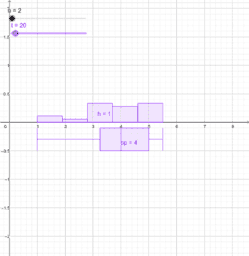Discover Central Limit Theorem Dice

Activity

DavidK

•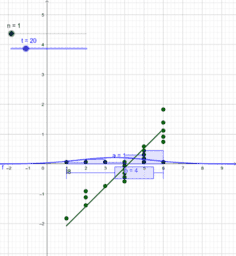Discover the Central Limit Theorem

Activity

DavidK

•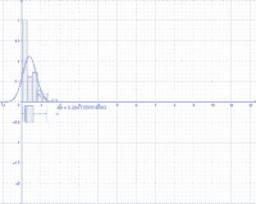Discover How to Assess Normality

Activity

DavidK

•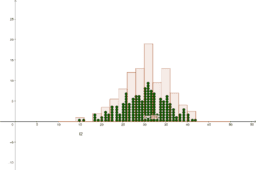Bell Shaped Data and the Empirical Rule

Activity

DavidK

•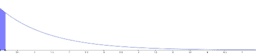Exponential Distribution CDF and Inverse

Activity

DavidK

•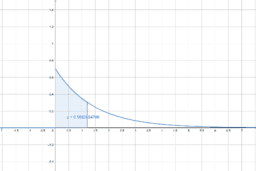Inverse Exponential Distribution

Activity

DavidK

•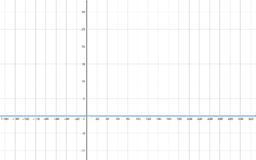Parameters of Exponential Distribution with Half-Life

Activity

DavidK

•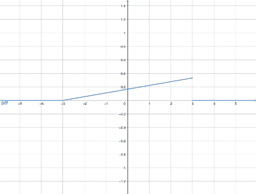Activity

DavidK